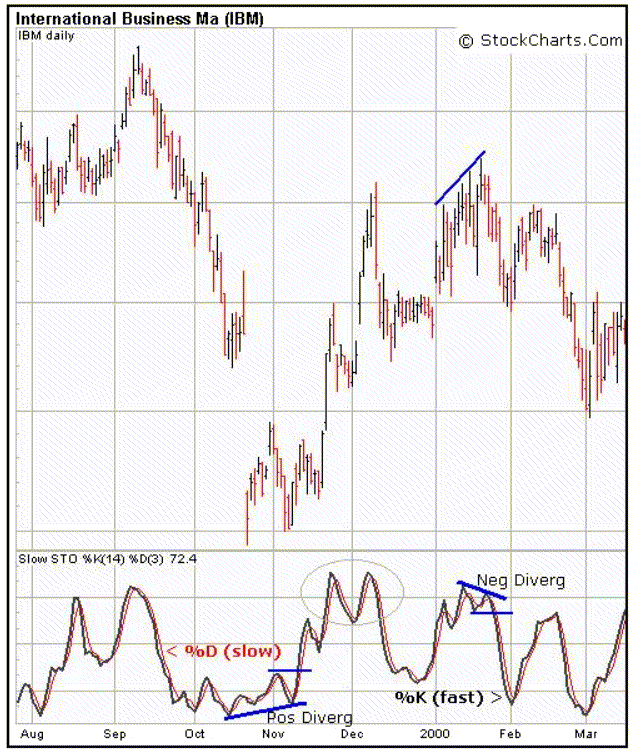## Stochastic Oscillator

The Stochastic Oscillator is a technical momentum indicator that compares a security’s closing price to its price range over a given time period, usually 14 periods. (1 period = 1 day for this example)  This indicator is calculated with the following formula:

%K = 100[(C – L14)/(H14 – L14)]

%D = Smoothed, 3 day simple moving average of %K

C = the most recent closing price

L14 = the low of the 14 previous trading sessions

H14 = the highest price traded during the same 14-day period

As an example, below is a table of daily highs, lows and closing prices for a particular index over 14 trading days.  On day 14, %K would be calculated as shown below where C=115.38, L14=109.13, and H14=119.94.%K for trading day 14 where the index closed at 115.38 equals 57.81.  This tells us that the index closed in the 58th percentile of the 14 day high/low range, or just above the mid-point.  Because %K is a ratio, it will fluctuate between 0 and 100.  A smoothed, 3-day simple moving average of %K is then plotted alongside to act as a trigger line, called %D.

Below is an example of a daily chart of IBM and its respective Stochastic Oscillator, courtesy of StockCharts.com.  One of the more conventional methods to leverage this indicator is to identify overbought and oversold situations by looking for divergences.  For example, the stochastic oscillator produced 2 solid signals for IBM between Aug-99 and Mar-00.  In Nov-99, a buy signal was given when the indicator formed a positive divergence and moved above 20 for the second time.  Note that the double top in Nov-Dec (gray circle) was not a negative divergence, and thus the stock continued higher after this formed.  In Jan-00, a sell signal was given when a negative divergence formed and the indicator dipped below 80 for the second time.